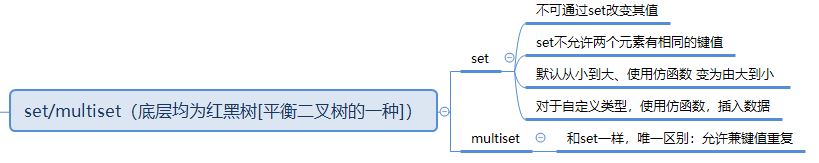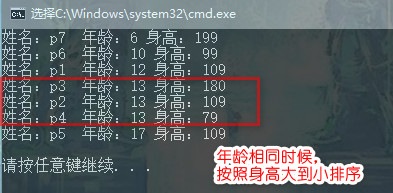​ 另外单独举一个完整示例。使用`STL` 模板的`set`容器，对自定义的数据类型，进行相应的插入和排序。具体使用了`set``multiset`进行举例子，他们的区别和联系，和使用自定义规则进行插入排序。以及二级排序的示范

[TOC]

## 简述：

​ 解决错误error C2678 类型方案

`1>c:\program files (x86)\microsoft visual studio 14.0\vc\include\xstddef(239): error C2678: 二进制“<”: 没有找到接受“const Person”类型的左操作数的运算符(或没有可接受的转换)`## 错误原因：

``````		// TEMPLATE STRUCT less
template<class _Ty = void>
struct less
{	// functor for operator<
typedef _Ty first_argument_type;
typedef _Ty second_argument_type;
typedef bool result_type;

constexpr bool operator()(const _Ty& _Left, const _Ty& _Right) const
{	// apply operator< to operands
return (_Left < _Right);
}
};``````

## 解决方案：

``set<Person, MyCompare> s;``

## 项目背景：## 思路架构：

• 创新自定义的`Person`数据类型

• 创建`Person`对象

• 创建仿函数**`MyCompare`**，

• 创建容器`s`

• `Person`对象插入`multiset`容器（为了演示二级排序，需要有重复的数值，故使用multiset）

• 打印容器`s`里面的结果

## 具体步骤：

``````#include <iostream>
using namespace std;
#include <set>  //set和multiset均使用此头文件
#include <string>

//自定义类型
class Person
{
public:
Person(string name, int age, int height):m_strname(name), m_nAge(age), m_nHeight(height){}
~Person(){}

public:
string m_strname;
int m_nAge;
int m_nHeight;

};

//利用仿函数 指定set容器的排序
class MyCompare
{
public:
bool operator()(Person p1, Person p2)
{
if (p1.m_nAge == p2.m_nAge)
{
return p1.m_nHeight > p2.m_nHeight;
}

return p1.m_nAge < p2.m_nAge;  //年龄从小到大排序
}

};

void printfSet(multiset<Person, MyCompare>& s)
{
for (multiset<Person, MyCompare>::iterator it = s.begin(); it != s.end(); it++)
{
cout << "姓名：" << it->m_strname << "  年龄：" << it->m_nAge << " 身高：" << it->m_nHeight << endl;
}
cout << endl;
}

int main()
{
Person p1("p1", 12, 109);
Person p2("p2", 13, 109);
Person p3("p3", 13, 180);
Person p4("p4", 13,  79);
Person p5("p5", 17, 109);
Person p6("p6", 10, 99);
Person p7("p7",  6, 199);

//set<Person> s;  //直接写，不知按照什么“大小规则”进行插入
//set<Person, MyCompare> s;   //加入仿函数， 使用“自定义规则”插入排序
multiset<Person, MyCompare> s;
s.insert(p1);
s.insert(p2);
s.insert(p3);
s.insert(p4);
s.insert(p5);
s.insert(p6);
s.insert(p7);

printfSet(s);

return 0;
}``````

## 运行演示：## 细节方面：

1. 先按照年龄排序，年龄由小到大
2. 若年龄相同，则按照身高由大到小排序
``````//利用仿函数 指定set容器的排序
class MyCompare
{
public:
bool operator()(Person p1, Person p2)
{
if (p1.m_nAge == p2.m_nAge)
{
return p1.m_nHeight > p2.m_nHeight;
}

return p1.m_nAge < p2.m_nAge;  //年龄从小到大排序
}

};``````

## 本次心得：